## RD Sharma Class 8 Solutions Chapter 17 Understanding Shapes III (Special Types of Quadrilaterals) Ex 17.2

These Solutions are part of RD Sharma Class 8 Solutions. Here we have given RD Sharma Class 8 Solutions Chapter 17 Understanding Shapes III Ex 17.2

Other Exercises

Question 1.
Which of the following statements are true for a rhombus ?
(i) It has two pairs of parallel sides.
(ii) It has two pairs of equal sides.
(iii) It has only two pairs of equal sides.
(iv) Two of its angles are at right angles.
(v) Its diagonals bisect each other at right angles.
(vi) Its diagonals are equal and perpendicular.
(vii) it has all its sides of equal lengths.
(viii) It is a parallelogram.
(x) It can be a square.
(xi) It is a square.
Solution:
(i) True
(ii) True
(iii) False (Its all sides are equal)
(iv) False (Opposite angles are equal)
(v) True
(vi) False (Diagonals are not equal)
(vii) True
(viii) True
(ix) True
(x) True (It is a rhombus)
(xi) False

Question 2.
Fill in the blanks, in each of the following, so as to make the statement true:
(i) A rhombus is a parallelogram in which ………..
(ii) A square is a rhombus in which ………..
(iii) A rhombus has all its sides of …….. length.
(iv) The diagonals of a rhombus each ………. other at ………. angles.
(v) If the diagonals of a parallelogram bisect each other at right angles, then it is a ………..
Solution:
(i) A rhombus is a parallelogram in which adjacent sides are equal.
(ii) A square is a rhombus in which one angle is right angle.
(iii) A rhombus has all its sides of equal length.
(iv) The diagonals of a rhombus bisect each other at right angles.
(v) If the diagonals of a parallelogram bisect each other at right angles, then it is a rhombus.

Question 3.
The diagonals of a parallelogram are not perpendicular. Is it a rhombus ? Why or why not ?
Solution:
By definition of a rhombus, its diagonals bisect each other at right angle.
So, the given parallelogram is not a rhombus.

Question 4.
Solution:
The diagonals of a quadrilateral are perpendicular to each.It is not always possible to be a rhombus. It can be of the diagonals bisect each other at right angles and if not, then it is not rhombus as shown in the figure given above:

Question 5.
ABCD is a rhombus. If ∠ACB = 40°, find ∠ADB.
Solution:
In rhombus ABCD, diagonals AC and BD intersect each other at O.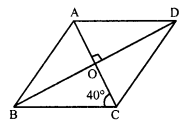∠ACB = 40°, we have to find ∠ADB.
BD || AD and AC.is its transversal..
Now in ∆AOD
∠OAD + ∠AOD + ∠ADO = 180° (Sum of angles of a triangle)
⇒ 40° + 90° + ∠ADO = 180°
⇒ 130° + ∠ADO = 180°
⇒ ∠ADO = 180° – 130° = 50°

Question 6.
If the diagonals of a rhombus are 12 cm and 16 cm, find the length of each side.
Solution:
In rhombus ABCD, diagonals AC and BD bisect each other at O at right angles.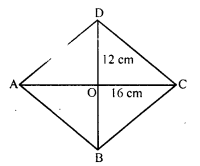AC = 16 cm, BD = 12 cm
AO = OC = $$\frac { 16 }{ 2 }$$ = 8 cm
BO = OD = $$\frac { 12 }{ 2 }$$ = 6 cm.
Now, in right angled ∆AOB,
AB² = AO² + BO² = (8)² + (6)² = 64 + 36 = 100 = (10)²
AB = 10 cm
Each side of rhombus = 10 cm

Question 7.
Construct a rhombus whose diagonals are of length 10 cm and 6 cm.
Solution:
(i) Draw a line segment AC =10 cm.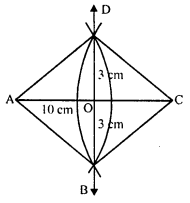(ii) Draw its perpendicular bisector and cut off OB = OD = 3 cm ($$\frac { 1 }{ 2 }$$ of 6 cm).
(iii) Join AB, BC, CD and DA.
Then ABCD is the required rhombus.

Question 8.
Draw a rhombus, having each side of length 3.5 cm and one of the angles as 40°.
Solution:
Steps of construction
(i) Draw a line segment AB = 3.5 cm.(ii) Draw a ray BX making an angle of 40° at B and cut off BC = 3.5 cm.
(iii) With centres C and A, and radius 3.5 cm. Draw arcs intersecting each other at D.
Then ABCD is a required rhombus.

Question 9.
One side of a rhombus is of length 4 cm and the length of an altitude is 3.2 cm. Draw the rhombus.
Solution:
(i) Draw a line segment AB = 4 cm.
(ii) At B, draw a perpendicular BX and cut off BL = 3.2 cm.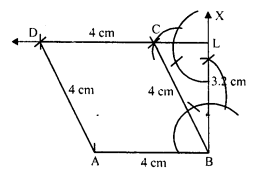(iii) At L, draw a line LY parallel to AB.
(iv) With centres B and radius 4 cm, draw an arc intersecting the line LY at C.
(v) With centre C cut off CD = 4 cm.
Then ABCD is the required rhombus.

Question 10.
Draw a rhombus ABCD if AB = 6 cm and AC = 5 cm.
Solution:
Steps of construction :
(i) Draw a line segment AB = 6 cm.
(ii) With centre A and radius 5 cm and with centre B and radius 6 cm, draw arcs intersecting each other at C.(iii) Join AC and BC.
(iv) Again with centres C and A and radius 6 cm, draw arcs intersecting each other at D.
Then ABCD is the required rhombus.

Question 11.
ABCD is a rhombus and its diagonals intersect at O.
(i) Is ∆BOC = ∆DOC ? State the congruence condition used ?
(ii) Also state, if ∠BCO = ∠DCO.
Solution:
In rhombus ABCD, diagonals AC and BD bisect each other at O.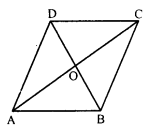(i) Now in ∆BOC and ∆DOC
OC = OC (Common)
BC = CD (Sides of rhombus)
OB = OC (Diagonals bisect each other at O)
∆BOC = ∆DOC (SSS. condition)
(ii) ∠BCO = ∠DCO

Question 12.
Show that each diagonal of a rhombus bisects the angle through which it passes:
Solution:
In rhombus ABCD, AC is its diagonal we have to prove that AC bisects ∠A and ∠C.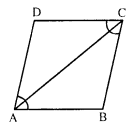AC = AC (Common)
AB = CD (Sides of a rhombus)
∠BAC = ∠DAC and ∠BCA = ∠DCA (c.p.c.t.)
Hence AC bisects the angle A.
Similarly, by joining BD, we can prove that BD bisects ∠B and ∠D.
Hence each diagonal of a rhombus bisects the angle through which it passes.

Question 13.
ABCD is a rhombus whose diagonals intersect at O. If AB = 10 cm, diagonal BD = 16 cm, find the length of diagonal AC.
Solution:
In rhombus ABCD,AB = 10 cm, diagonal BD = 16 cm.
Draw diagonal AC which bisects BD at O at right angle.
BO = OD = 8 cm and AO = OC.
Now in ∆AOB,
AB² = AO² + BO²
⇒ (10)² = AO² + (8)²
⇒ 100 = AO² – 64
⇒ AO² = 100 – 64 = 36 = (6)²
AO = 6.
AC = 2AO = 2 x 6 = 12 cm

Question 14.
The diagonals of a quadrilateral are of lengths 6 cm and 8 cm. If the diagonals bisect each other at right angles, what is the length of each side of the quadrilateral ?
Solution:
In a quadrilateral ABCD, diagonals AC and BD bisect each other at right angles.
AC = 8 cm and BD = 6 cm.AO = OC = 4 cm and BO = OD = 3 cm
Now, in right ∆AOB,
AB² = AO² + BO² = (4)² + (3)² = 16 + 9 = 25 = (5)²
AB = 5 cm
Hence each side of quadrilateral will be 5 cm.

Hope given RD Sharma Class 8 Solutions Chapter 17 Understanding Shapes III Ex 17.2 are helpful to complete your math homework.

If you have any doubts, please comment below. Learn Insta try to provide online math tutoring for you.